# Portfolio Optimization (Research & Algorithm)

One of the main advantages of the QuantConnect platform is the ability to move seamlessly between the research and production environments with minimal or no code changes required.

In this post, we will go through an analysis on several portfolio optimization techniques performed using the QuantConnect Jupyter Notebook. At the end, you will find the full project (backtesting algorithm and research notebook) ready for you to clone and work with! The purpose of this project is to provide users with a powerful workflow in QuantConnect, performing your research in the notebook (changing assets, the historical period, lookback windows, etc.) and then backtesting your conclusions and ideas in the Algorithm Framework.

### Portfolio Optimization Methods

Portfolio Optimization refers to the process of choosing the best possible allocation among certain assets (e.g. individual stocks, asset classes) according to some specific objective (e.g. minimize risk, maximize risk-adjusted returns, achieve risk parity) and subject to optional constraints (e.g. long-only positions, fully invested).

Let's briefly present the different objective functions that will be part of our research.

#### Equally-Weighted Portfolio (benchmark)

A portfolio in which all assets receive the exact same allocation. This is not an optimized portfolio, but we will be using it as a benchmark.

#### Maximize Portfolio Return (disregard volatility)

Without any boundaries on weights or constraints on target portfolio volatility, this is a very simple function that will allocate 100% to the asset with the highest expected return.

#### Minimize Portfolio Volatility (disregard return)

Without any constraint around target portfolio return, this optimization will find the allocation that, given the data, generates the portfolio with the lowest volatility (measured by estimated standard deviation).

#### Maximize Portfolio Sharpe Ratio (assuming a risk-free rate of 0%)

The Sharpe Ratio measures the average return earned (in excess of the risk-free rate) per unit of total risk. Its calculation is simply the portfolio expected return divided by the portfolio expected volatility (measured by estimated standard deviation).

#### Maximize Portfolio Sortino Ratio

Similar to the Sharpe Ratio, the Sortino Ratio is another measure of the risk-adjusted returns of an investment that only factors in the downside, or negative volatility, rather than the total volatility. There are several variations of this ratio, but in its most basic form its calculation is the portfolio expected return divided by the portfolio expected downside volatility (standard deviation of negative returns).

#### Risk Parity Portfolio

With the objective to offer equity-like returns and bond-like risk, this approach defines a well-diversified portfolio where all assets have the same marginal contribution to the total risk of the portfolio. In this sense, a Risk Parity portfolio is an equally weighted portfolio, where the weights refer to risk rather than dollar amount invested in each asset, resulting in a heavier allocation to the lower volatility assets. Roughly speaking, the approach is similar to creating a minimum variance portfolio subject to the constraint that each asset contributes equally to the overall portfolio volatility (as we will see later, a risk parity portfolio lies between an equally-weighted portfolio and a minimum variance portfolio). In our implementation, we will be using the nice formulation proposed by Spinu which is strictly convex.

### Preliminary Analysis

#### The Assets

We will be constructing portfolios using the following ETFs:

• IEF: iShares 7-10 Year Treasury Bond
• TLT: iShares 20+ Year Treasury Bond
• GLD: SPDR® Gold Shares
• SPY: SPDR® S&P 500® ETF Trust
• QQQ: Invesco QQQ Trust (Nasdaq-100 Index)

With the following Correlation Matrix (based on historical daily returns from 2006 to April 2020):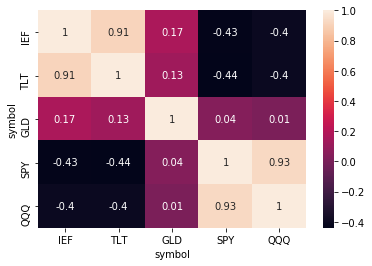As we would expect, Bond (IEF/TLT) and Equity (SPY/QQQ) indices  groups show high positive correlation within themselves, and negative correlation against each other. Also, Gold seems to have relative low correlation against both Bonds and Equities.

#### The Mean-Variance Space And Efficient Frontier

Using historical daily returns from 2006 to April 2020, we are now going to take these assets and construct all the optimized portfolios defined above, which we will visualize in the Mean-Variance space (Annualized Volatility/Annualized Return) along with each individual asset and 5,000 simulated portfolios.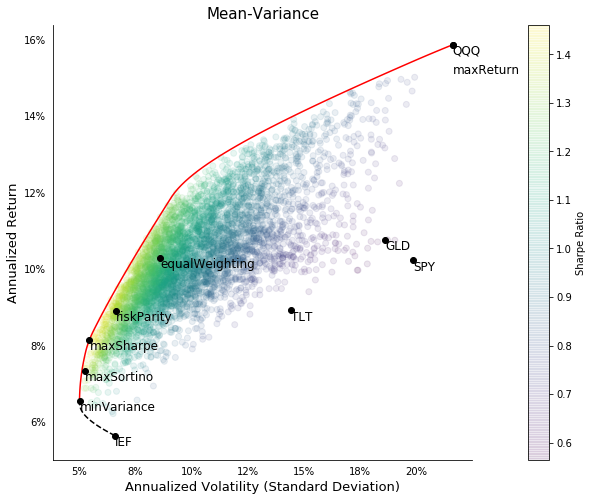There is a lot going up there so let's try summarize the main takeaways from this visualization. Note the portfolios above have the constraints of being long-only (weights lie between 0 and 1) and fully invested (weights sum to 1).

• The Efficient Frontier extends from the Minimum Variance portfolio to the asset with the highest return (QQQ), wrapping inside all the possible portfolios. Observe how IEF (the single asset with the lowest volatility) and all the portfolios lying on the dotted black line are not efficient portfolios since we can achieve higher returns for the same level of risk by changing the allocation.
• Every optimized portfolio seems to offer better risk-adjusted returns than any of the individual assets alone.
• Among the different optimized portfolios, only Minimum Variance and Maximum Sharpe Ratio lie on the Efficient Frontier and are therefore efficient portfolios (i.e. there is no other possible combination of weights that yields higher return for that particular level of risk).
• Risk Parity lies between Minimum Variance and Equal-Weighting.

#### Portfolio Weights And Relative Risk Contributions

It is interesting to see the impact of the different allocations to the distribution of risk in the portfolio. The Relative Risk Contribution (RRC) shows how much each asset risk contributes to the overall risk in the portfolio, and it's the result of the assets volatilities, the correlations with each other, and of course their weights in the portfolio.

We are especially interested in the comparison between Equal-Weighting, Minimum Variance and Risk Parity. Let's compare the weights and relative risk contribution of each asset in the portfolios, alongside their individual volatilities.• Only Risk Parity generates a portfolio of equally-weighted risk contributions, whereas Equal-Weighting yields a risk distribution that depends entirely on the assets volatilities and their correlations.
• Unsurprisingly, Minimum Variance heavily allocates to IEF (the asset with the lowest volatility), and the remaining to SPY (with negative correlation to IEF) and very little to GLD (very low correlation to both IEF and SPY), resulting in almost all the risk concentrated in IEF.

### Walk-Forward Analysis Of Optimized Portfolios

The above analysis was done using historical data from the entire period 2006-April 2020. However, the underlying characteristics of the assets (mean returns, volatility, correlations, etc.) are changing so in order to understand how these techniques would have behaved over time we need to perform a walk-forward analysis. Every month, we are going to rebalance our portfolios for the next month, allocating the optimal weights calculated based on a lookback window of 3 months.

#### Monthly Portfolio Weights

Before looking at the performance of each portfolio, we want to look at the time series of monthly weights to get a sense for how each technique allocates among the assets depending on the period.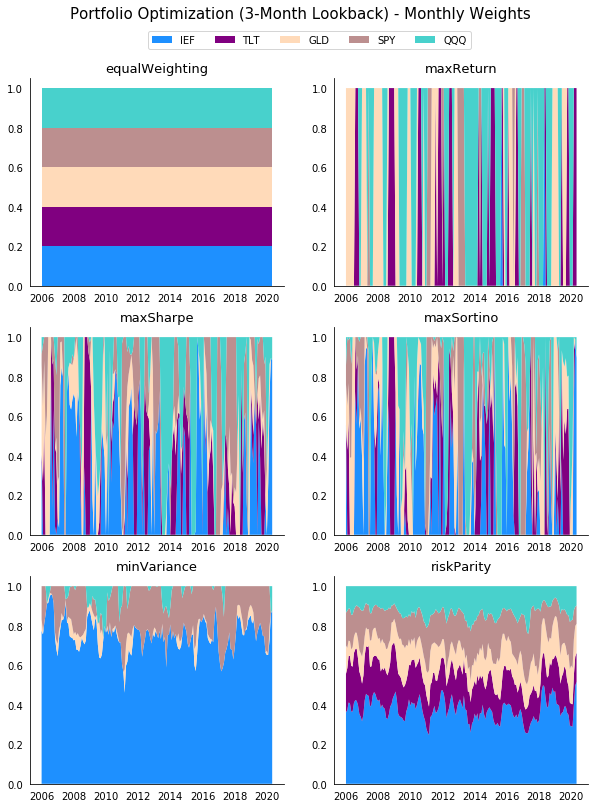• Maximum Return is the least diversified portfolio over time, basically jumping between QQQ and SPY, and a few times TLT.
• Maximum Sharpe and Sortino Ratios are also very unstable, but at least seem to allocate to several assets at a time.
• Finally, notice how stable and well-diversified Risk Parity is. Minimum Variance also doesn't surprise much over time, but it's a far less diversified portfolio with most of the allocation in IEF.

#### Cumulative Returns And Drawdown

Now it's time to see the performance over time. Below we can see the time series of cumulative returns and drawdown for each of the assets alone.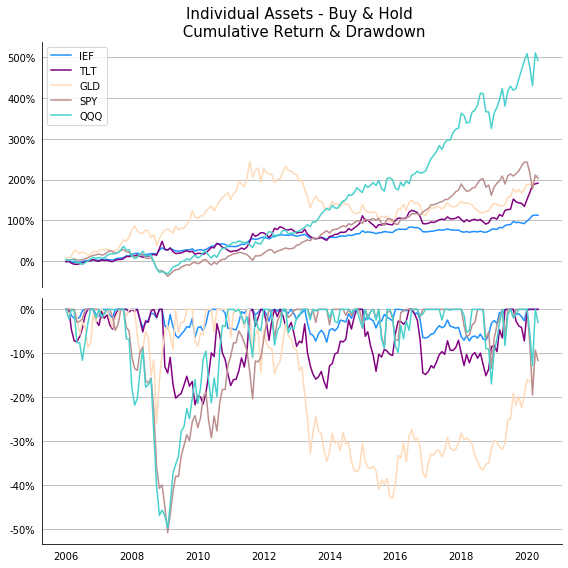• QQQ is by far the asset with the highest cumulative return, but also with the highest volatility and maximum drawdown. On the other extreme, IEF returns 5 times less during the 14 year period, but of course with the lowest risk.
• SPY seems to show similar volatility and drawdown to QQQ, however the returns over time are more than half.
• TLT and GLD have similar returns to SPY, though as expected TLT presents lower volatility throughout. Interestingly, GLD has drawdowns that do not necessarily coincide in time with those of SPY and the rest, which will come in handy when building a diversified portfolio as we will see later.

And now for our optimized portfolios!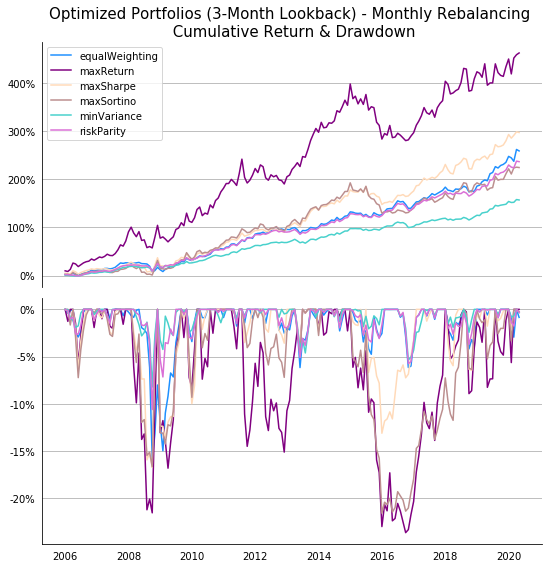• Similar to the assets performance, there are clear differences in terms of risk/return characteristics. The strategy with the highest cumulative return is Maximum Return (in which QQQ dominates) and the one with the lowest is Minimum Variance (dominated by IEF).
• In between Maximum Return and Minimum Variance, the rest of the strategies seem to end on a similar point. However, the drawdowns and overall volatility is clearly not the same.

#### Risk-Reward Profiles

Let's visualize all this information in a more clear way. Below there are three scatter plots with different Risk-Reward profiles for both the assets and the portfolios: Return-Volatility, Return-Max Drawdown and Sharpe Ratio-Max Drawdown.• In all these plots, the sweet spot is always in the northwest quadrant. Minimum Variance and Risk Parity are the portfolios with the highest risk-adjusted returns (1.4 SR) and the lowest possible drawdown.
• Risk Parity offers returns on par with SPY by taking risks similar to IEF. Once again, the performance of Risk Parity is between Equal-Weighting and Minimum Variance.
• Maximum Return, a portfolio that we recall allocates 100% to the highest momentum stock every month, yields a cumulative return very close to QQQ (the highest growth asset) and with similar annualized volatility, but it manages to reduce the drawdown of QQQ by half (from 50% to 24%) by switching allocation in key moments.
• The choice of investment would really depend on the investor's risk-aversion, but it's at least clear that the optimized portfolios outperform the individual assets on a risk-adjusted bases and in some cases even on pure returns.

#### Multiple Lookback Periods

To finish our walk-forward analysis we need to test multiple lookback periods in order to assess the stability of the different methods over time. We're going to run all the strategies rebalancing every month using 1, 2, 3, 6, 9 and 12-month lookbacks for the optimization, and visualize the results on the Return-Volatility space.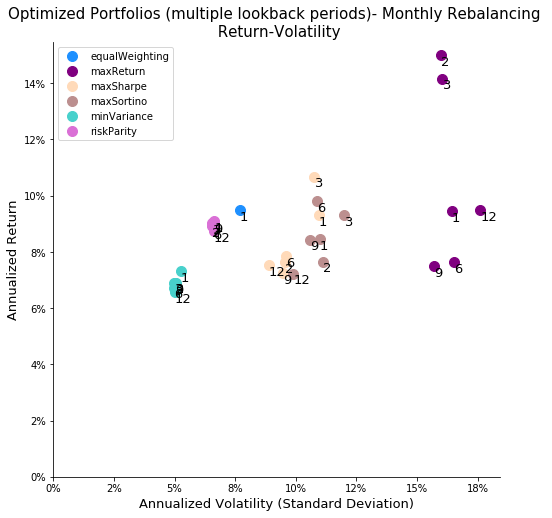• This plot illustrates the importance of stability. The performance of Minimum Variance and Risk Parity barely deviates when using different lookback periods, however the other three methods are all over the place.
• One reason for this is that optimizations that only use the second moment (variance) are more stable than the ones including the mean returns.
• Also, the way we defined Maximum Return means that it only picks one asset at a time depending on past performance, and therefore the lookback period plays an exaggerated role here.

### Clone The Algorithm

Below you will find the complete code for backtesting and research in QuantConnect!

#### PORTFOLIO OPTIMIZATION SYSTEM (Research & Algorithm)

In the `main.py` script you will find some user-defined inputs to perform the different optimizations:

1) Input a list of tickers

``tickers = ['IEF', 'TLT', 'GLD', 'SPY', 'QQQ']``

2) Select the objective function (options are: equalWeighting, maxReturn, minVariance, meanVariance, maxSharpe, maxSortino, riskParity)

``objectiveFunction = 'riskParity'``

3) Choose the number of trading days for the lookback period

``lookbackOptimization = 63``

4) Select the logic for rebalancing period (options are: Expiry.EndOfDay, Expiry.EndOfWeek, Expiry.EndOfMonth, Expiry.EndOfQuarter, Expiry.EndOfYear)

``rebalancingPeriod = Expiry.EndOfMonth``

### Conclusions

In this post we presented some preliminary research on portfolio optimization, including walk-forward analysis on multiple lookback windows:

• Using a seemingly diversified basket of ETFs from different asset classes (Equities, Bonds and Gold), we built some interesting portfolios applying classic optimization techniques.
• The results (2006-April 2020) show that we were able to construct portfolios offering better risk-adjusted returns than the individual assets. Also, Minimum Variance and Risk Parity beat our benchmark (Equal-Weighing portfolio) on a risk-adjusted basis achieving a 1.4 Sharpe Ratio (vs 1.2) and a much lower drawdown.
• Finally, we saw how the choice of the lookback horizon can be a major factor in the performance of optimizations that include the first moment (mean), but barely affects the ones solely based on variance.

Do you have a strategy of your own that you would like to backtest and automate? Learn about our consulting services and get in touch at

Found this post useful? You can support this site by making a donation using the link below!## 1 comment

• Mark

Really nice work. Thanks for sharing!